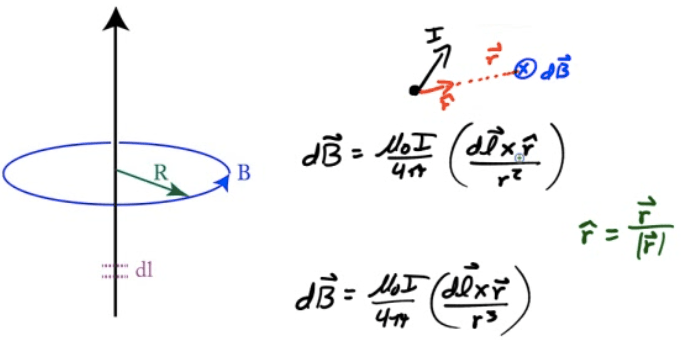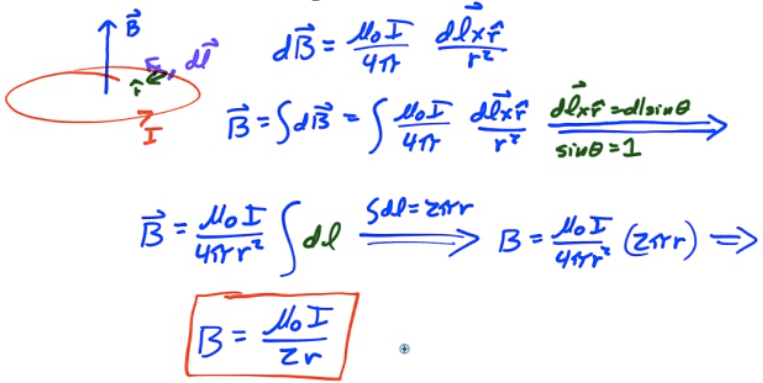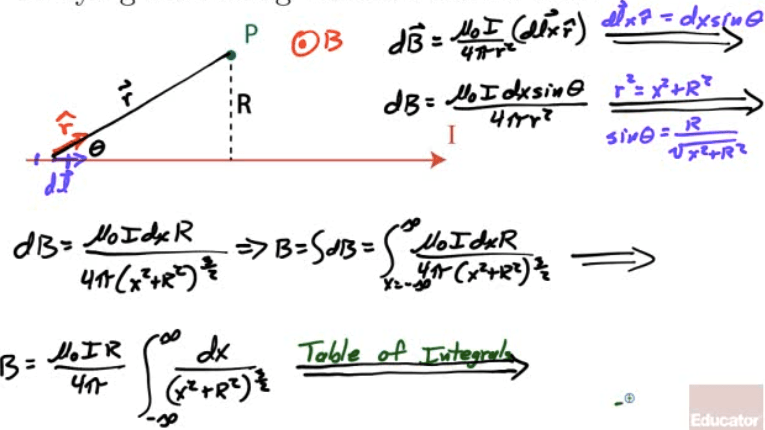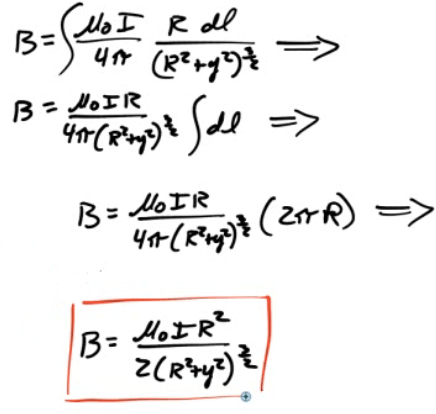# Biot-Savart Law

• A "brute force" method of finding the magnetic field due to a length of current-carrying wire# Example 1: Magnetic Field due to a Current Loop

• Derive the magnetic field due to a current loop at the center of the loop# Example 2: Magnetic Field due to Long Straight Current-Carrying Wire

• Derive the magnetic field strength at a point P located a distance R from an infinitely long current-carrying wire using the Biot-Savart Law# Example 3: Magnetic Field due to A Current Loop

• Derive the magnetic field due to a current loop at a point out of the plane of the loop but centered on the loop's axis as shown